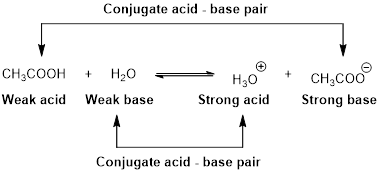# Which of the following ions or compounds has the strongest conjugate base? Briefly explain your choice. (a) HSO 4 − (b) CH 3 CO 2 H (c) HOCl### Chemistry & Chemical Reactivity

9th Edition
John C. Kotz + 3 others
Publisher: Cengage Learning
ISBN: 9781133949640

#### Solutions

Chapter
Section### Chemistry & Chemical Reactivity

9th Edition
John C. Kotz + 3 others
Publisher: Cengage Learning
ISBN: 9781133949640
Chapter 16, Problem 17PS
Textbook Problem
1 views

## Which of the following ions or compounds has the strongest conjugate base? Briefly explain your choice. (a) HSO4− (b) CH3CO2H (c) HOCl

Interpretation Introduction

Interpretation:

The strongest conjugate base has to be identified from the given ions or compounds.

Concept introduction:

An equilibrium constant (K) is the ratio of concentration of products and reactants raised to appropriate stoichiometric coefficient at equlibrium.

For the general acid HA,

HA(aq)+H2O(l)H3O+(aq)+A(aq)

The relative strength of an acid and base in water can be also expressed quantitatively with an equilibrium constant as follows:

Ka=[H3O+][A][HA]

An equilibrium constant (K) with subscript a indicate that it is an equilibrium constant of an acid in water.

For the general base B,

B(aq)+H2O(l)BH(aq)+OH(aq)

The relative strength of an acid and base in water can be also expressed quantitatively with an equilibrium constant as follows:

Kb=[BH][OH][B]

An equilibrium constant (K) with subscript b indicate that it is an equilibrium constant of base in water.

Bronsted –Lowry conjugated acid-Base pairs:

When an acid is dissolved in water, the acid (HA) donates a proton to water to form a new acid (conjugated acid) and a new base (conjugated base).The pair of an Acid –Base differs by a proton called conjugated Acid-Base pair.

### Explanation of Solution

Reason for the correct statement

For the general acid HA,

HA(aq)+H2O(l)H3O+(aq)+A(aq)

Ka=[H3O+][A][HA]

A large value of Ka indicates that the ionization product is strongly favoured because the product [H3O+][A] is more than the equilibrium concentration of the strong acid [HA].

A conjugate acid-base pair contains two compounds that differ only by a hydrogen ion and a charge of +1. Thus, HA and A are conjugate acid-base pair. In this pair, A is a conjugate base of an acid HA

### Still sussing out bartleby?

Check out a sample textbook solution.

See a sample solution

#### The Solution to Your Study Problems

Bartleby provides explanations to thousands of textbook problems written by our experts, many with advanced degrees!

Get Started

Find more solutions based on key concepts
T-cells are immune cells that "read" and "remember" chemical messages to identify future invaders. T F

Nutrition: Concepts and Controversies - Standalone book (MindTap Course List)

What are the chemical differences between DNA and RNA?

Biology: The Dynamic Science (MindTap Course List)

Assign an IUPAC name to each of the following alcohols.

General, Organic, and Biological Chemistry

_______ is the arrival of pollen on a receptive stigma.

Biology: The Unity and Diversity of Life (MindTap Course List)

The Doppler equation presented ill the text is valid when the motion between the observer and the source occurs...

Physics for Scientists and Engineers, Technology Update (No access codes included)